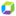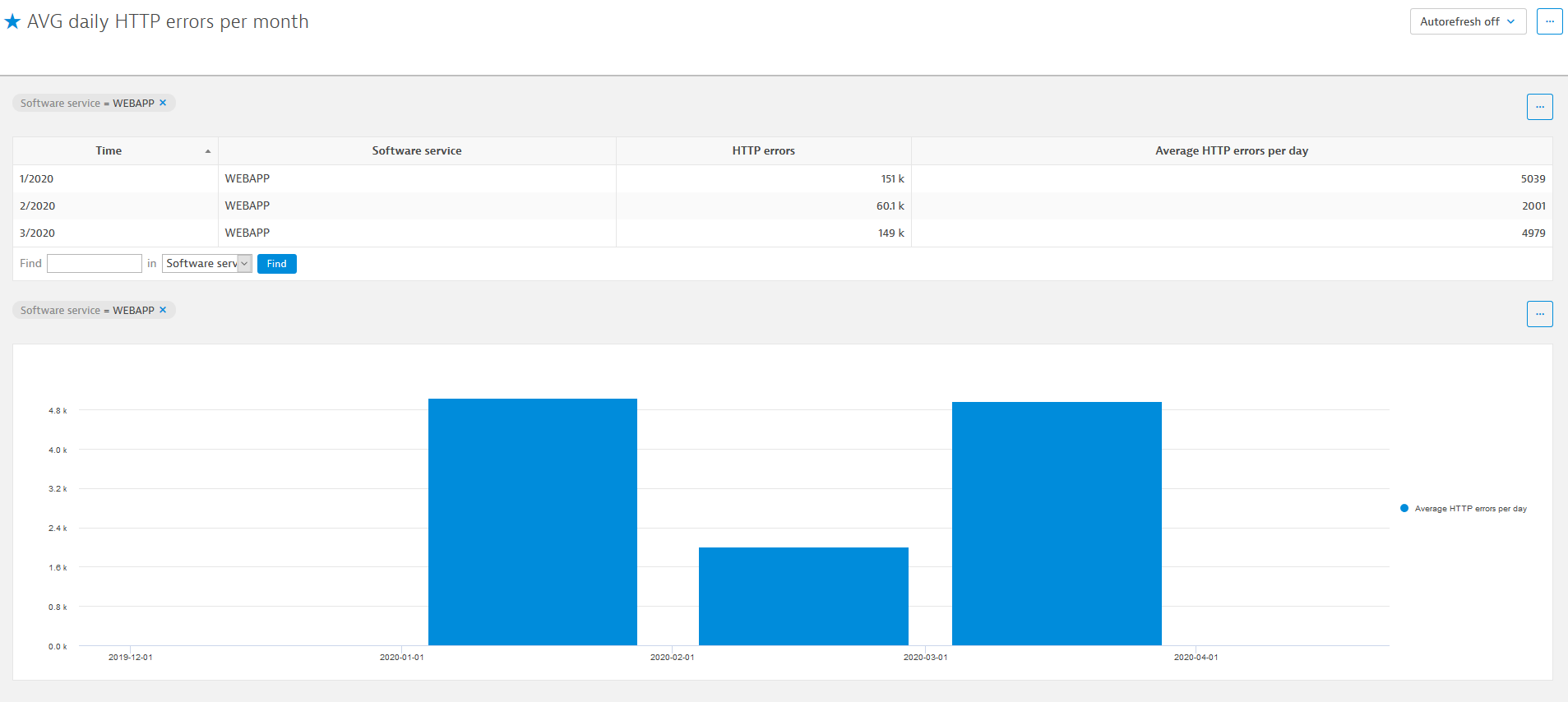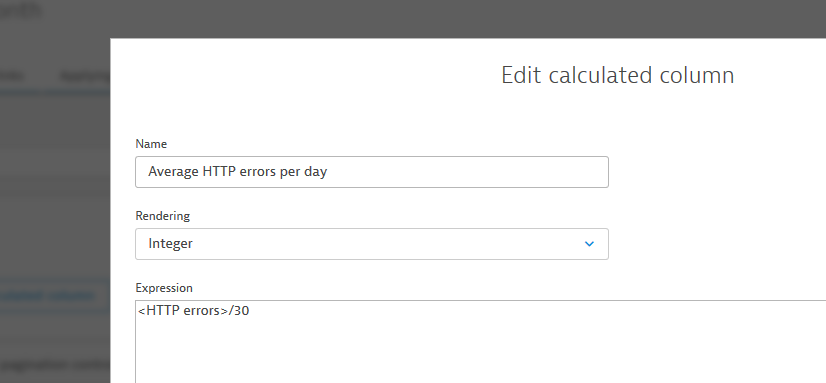cancel
Showing results for
Show  only  | Search instead for
Did you mean:

## How to change aggregation of metrics in NAM?Pro

For Example, I look at the chart of HTTP 404 error using last 3 month with monthly resolution.

So what I see are 3 bar, where each bar shows the SUM of HTTP 404 error for that month.

What if I want each bar to show AVERAGE HTTP 404 error per day instead of SUM?

I know I can knew the answer by change the monthly resolution to daily resolution to see 30/60/90 bar. But right now I need to achieve this by 3 bar only.

Best Regards,

Wai Keat

2 REPLIES 2Dynatrace Pro

If I understand you correctly, you need to divide the number you have by number of days in a month. If this is correct, then it looks like a custom metric definition.Dynatrace Pro

Hello,

I like Kris idea with calculated metrics, and here's example simple implementation:Average HTTP errors per day metric is just a calculated column, my formula is very simple, it's just dividing sum of errors by 30, as shown below:Whole report's definition in attachment: AVG daily HTTP errors per month.zip